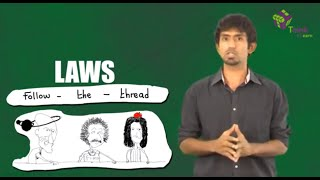# Motion In Physics

The concept of motion in physics is one of the important topics under Classical Mechanics. Did you know that everything in the universe is always moving? Even if you are completely still, you still belong to the earth which is continuously moving about its axis and around the sun. Motion means change in the position of an object with reference to time.## What is Motion in Physics?

In physics, the motion is the change in position of an object with respect to its surroundings in a given interval of time. The motion of an object with some mass can be described in terms of the following:

• distance
• displacement
• speed
• velocity
• time
• acceleration## Types of Motion in Physics

Motion of an object depends on the type of force acting on the body. Examples of different kinds of motion are given below.

• Translational – It is the type, where an object moves along a path in any of the three dimensions.
• Rotational – It is the type, where an object moves along a circular path about a fixed axis.
• Linear – It is a type of translational motion where the body moves in a single direction along a single dimension.
• Periodic – It is the type of motion that repeats itself after certain intervals of time
• Simple Harmonic  It is the type of motion like that of a simple pendulum where a restoring force acts in the direction opposite to the direction of motion of the object. This restoring force is proportional to the displacement of the object from mean position.
• Projectile It is the type of motion which has a horizontal displacement as well as vertical displacement.
• Oscillatory  It is the type of motion which is repetitive in nature within a time frame. If it is mechanical in nature it is called vibration.

## Laws of Motion

Newton’s Laws of Motion laid the foundation for classical mechanics today. Although subject to minor limitations, these laws of motion are valid everywhere and are therefore used. The laws are given as stated below in a brief description

• First Law: Any object will continue to remain in its existing state of motion or rest unless a net external force acts on it.
• Second Law: If an object has a certain mass, greater the mass of this object, greater will the force required be to accelerate the object. It is represented by the equation F = ma, where ‘F’ is the force on the object, ‘m’ is the mass of the object and ‘a’ is the acceleration of the object.
• Third Law: For every action, there is an equal and opposite reaction.Motion is an important concept in Physics which can be better understood by applying conceptual knowledge to solve problems. Stay tuned with BYJU’S and learn various interesting physics topic with the help of engaging video lessons.

I love it

2. Yash

Yes,very nice explain action in BYJUS and I already registered in BYJUS

3. lizzy

this is very helpful thank you